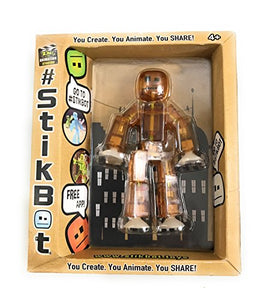# Stikbot, Translucent Brown Stikbot Action Figure, 3 Inches

Vendor
Zing
Regular price
Sold out
Sale price
\$25.79
Quantity must be 1 or more

• S

• t

• i

• k

• b

• o

• t

• ,

• T

• r

• a

• n

• s

• l

• u

• c

• e

• n

• t

• B

• r

• o

• w

• n

• S

• t

• i

• k

• b

• o

• t

• A

• c

• t

• i

• o

• n

• F

• i

• g

• u

• r

• e

• ,

• 3

• I

• n

• c

• h

• e

• s

Stikbot, Translucent Brown Stikbot Action Figure